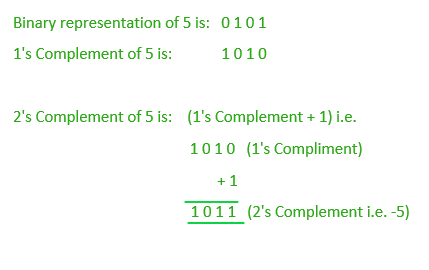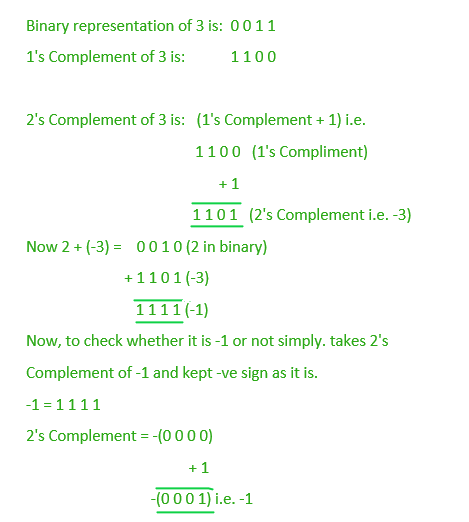# Subtraction of two numbers using 2’s Complement

Given two numbersand. The task is to subtractfromby using 2’s Complement method.

Note: Negative numbers represented as 2’s Complement of Positive Numbers.

For example, -5 can be represented in binary form as 2’s Compliment of 5. Look at the image below:Examples:

```Input : a = 2, b = 3
Output : -1

Input : a = 9, b = 7
Output : 2
```

## Recommended: Please try your approach on {IDE} first, before moving on to the solution.

To subtractfrom. Write the expression (a-b) as:

```(a - b) = a + (-b)
```

Now (-b) can be written as (2’s complement of b). So the above expression can be now written as:

```(a - b) = a + (2's complement of b)
```

So, the problem now reduces to “Addto the 2’s complement of“. Below image illustrates the above method of subtraction for the first example where a = 2 and b = 3.Below is the implementation of above method:

## C++

 `#include ` `using` `namespace` `std; ` ` `  `// function to subtract two values ` `// using 2's complement method ` `int` `Subtract(``int` `a, ``int` `b) ` `{ ` `    ``int` `c; ` ` `  `    ``// ~b is the 1's Complement of b  ` `    ``// adding 1 to it make it 2's Complement ` `    ``c = a + (~b + 1); ` ` `  `    ``return` `c; ` `} ` ` `  `// Driver code ` `int` `main() ` `{ ` `    ``int` `a = 2, b = 3; ` ` `  `    ``cout << Subtract(a, b)<

## Java

 `class` `GFG  ` `{ ` ` `  `// function to subtract two values ` `// using 2's complement method ` `static` `int` `Subtract(``int` `a, ``int` `b) ` `{ ` `    ``int` `c; ` ` `  `    ``// ~b is the 1's Complement ` `    ``// of b adding 1 to it make  ` `    ``// it 2's Complement ` `    ``c = a + (~b + ``1``); ` ` `  `    ``return` `c; ` `} ` ` `  `// Driver code ` `public` `static` `void` `main(String[] args) ` `{ ` `    ``int` `a = ``2``, b = ``3``; ` `     `  `    ``System.out.println(Subtract(a, b)); ` `     `  `    ``a = ``9``; b = ``7``; ` `    ``System.out.println(Subtract(a, b)); ` `} ` `} ` ` `  `// This code is contributed  ` `// by ChitraNayal `

## Python3

 `# python3 program of subtraction of ` `# two numbers using 2's complement . ` ` `  `# function to subtract two values ` `# using 2's complement method ` `def` `Subtract(a,b): ` `     `  `    ``# ~b is the 1's Complement of b  ` `    ``# adding 1 to it make it 2's Complement ` `    ``c ``=` `a ``+` `(~b ``+` `1``) ` `    ``return` `c ` ` `  `# Driver code ` `if` `__name__ ``=``=` `"__main__"` `: ` ` `  `    ``# multiple assignments ` `    ``a,b ``=` `2``,``3` `    ``print``(Subtract(a,b)) ` ` `  `    ``a,b ``=` `9``,``7` `    ``print``(Subtract(a,b)) `

## C#

 `// C# program of subtraction of ` `// two numbers using 2's complement ` `using` `System; ` ` `  `class` `GFG ` `{ ` `// function to subtract two values ` `// using 2's complement method ` `static` `int` `Subtract(``int` `a, ``int` `b) ` `{ ` `    ``int` `c; ` ` `  `    ``// ~b is the 1's Complement ` `    ``// of b adding 1 to it make  ` `    ``// it 2's Complement ` `    ``c = a + (~b + 1); ` ` `  `    ``return` `c; ` `} ` ` `  `// Driver code ` `static` `void` `Main() ` `{ ` `    ``int` `a = 2, b = 3; ` `     `  `    ``Console.WriteLine(Subtract(a, b)); ` `     `  `    ``a = 9; b = 7; ` `    ``Console.WriteLine(Subtract(a, b)); ` `} ` `} ` ` `  `// This code is contributed  ` `// by mits `

## PHP

 ` `

Output:

```-1
2
```

My Personal Notes arrow_drop_upCheck out this Author's contributed articles.

If you like GeeksforGeeks and would like to contribute, you can also write an article using contribute.geeksforgeeks.org or mail your article to contribute@geeksforgeeks.org. See your article appearing on the GeeksforGeeks main page and help other Geeks.

Please Improve this article if you find anything incorrect by clicking on the "Improve Article" button below.

Article Tags :

1

Please write to us at contribute@geeksforgeeks.org to report any issue with the above content.